• 脉冲神经网络最基本的神经元模型之一SRM模型的前世今生，英文原版
• 首先介绍各类常用的脉冲神经元模型以及前馈和循环型脉冲神经网络结构;然后介绍脉冲神经网络的时间编码方式,在此基础上,系统地介绍脉冲神经网络的学习算法,包括无监督学习和监督学习算法,其中监督学习算法按照梯度...
• 脉冲神经网络亲测运行实例，Python版本的，正确不用调，所用的神经元模型为IF模型，进行STDP无监督学习，数据集为MNIST，可供学习。
• 脉冲神经网络 神经元模型-Izhikevich模型 一 原理 之前的两篇博客分别介绍了HH模型和IF模型，其中HH模型过于复杂，不适合大规模仿真；IF模型过于简答，脉冲发射模式过于单一。因此，在2003年，Eugene M. Izhikevich...
脉冲神经网络 神经元模型-Izhikevich模型
一 原理
之前的两篇博客分别介绍了HH模型和IF模型，其中HH模型过于复杂，不适合大规模仿真；IF模型过于简答，脉冲发射模式过于单一。因此，在2003年，Eugene M. Izhikevich提出了Izhikevich模型，简化HH模型同时具有大量的发射模式。
1.模型
通过对HH模型进行分岔分析，并结合IF模型的计算效率，Izhikevich提出了二维脉冲神经元模型：

d

V

/

d

t

=

0.04

V

2

+

5

V

1

40

−

U

+

I

\mathrm{d}V/\mathrm{d}t = 0.04V^2+5V_140-U+I

d

U

/

d

t

=

a

(

b

V

−

U

)

\mathrm{d}U/\mathrm{d}t=a(bV-U)

如果神经元的膜电位

V

≥

V\geq

30mV,辅助的复位机制为：

V

⟵

V \longleftarrow

c

U

⟵

U \longleftarrow

U+d
2.参数
参数含义V膜电位U恢复变量，用来代替生理模型中激活的K离子电流和失活的Na离子电流，实现对膜电位V的负反馈a恢复变量U的时间尺度，a越小，恢复的越慢b恢复变量U依赖膜电位V的阈值下随机波动的敏感程度c发放脉冲后，V的复位值d发放买抽，U的复位值
二 代码
1. 单神经元
a=0.02;
b=0.2;
r=rand(1);
c=-6+15*r^2;
d=8-6*r^2;
V=-65;
u=b*V;
VF=[];
tau = 0.5
tspan=100:tau:1000;
VT=30;
for t=tspan
if(t>VT)
I=10+(0.015*(t-VT));
else
I=10;
end
V=V+0.25*(0.04*V^2+5*V+140-u+I);
u=u+0.25*a*(b*V-u)

if V>30
VF(end+1)=30;
V=c;
u=u+d;
else
VF(end+1)=V;
end
end
plot(tspan,VF);
set(gca,'FontSize',25);
xlabel('Time/ms','FontSize',25);ylabel('Membrane voltage/mV','FontSize',25);

2. 多神经元
按照Izhikevich论文中给的案例，兴奋型神经元800个，抑制型神经元200个（4:1）
%Created by Eugene M. Izhikevich, February 25, 2003
% Excitatory neurons Inhibitory neurons
Ne=4;
Ni=1;
re=rand(Ne,1);
ri=rand(Ni,1);
a=[0.02*ones(Ne,1); 0.02+0.08*ri];
b=[0.2*ones(Ne,1); 0.25-0.05*ri];
c=[-65+15*re.^2; -65*ones(Ni,1)];
d=[8-6*re.^2; 2*ones(Ni,1)];
S=[0.5*rand(Ne+Ni,Ne), -rand(Ne+Ni,Ni)];
v=-65*ones(Ne+Ni,1);   % Initial values of v
u=b.*v;                % Initial values of u
firings=[];            % spike timings
for t=1:1000           % simulation of 1000 ms
I=[5*randn(Ne,1);2*randn(Ni,1)];  % thalamic input
fired=find(v>=30);                % indices of spikes
firings=[firings; t+0*fired,fired];
v(fired)=c(fired);
u(fired)=u(fired)+d(fired);
I=I+sum(S(:,fired),2);
v=v+0.5*(0.04*v.^2+5*v+140-u+I);   % step 0.5 ms
v=v+0.5*(0.04*v.^2+5*v+140-u+I);   % for numerical
u=u+a.*(b.*v-u);                   % stability
end
plot(firings(:,1),firings(:,2),'.');


参考文献
Izhikevich, E. M. Simple model of spiking neurons. IEEE Trans Neural Netw, 2003 (14): 1569-1572.https://www.cnblogs.com/Flltju/p/10624693.html
展开全文• 脉冲神经网络的一个训练方法，基于PYTHON3写的，可以直接运行，神经元模型采用简化的脉冲响应模型。采用ASA训练算法
• 脉冲神经网络 神经元模型-IF模型...IF模型仅考虑HH神经元模型中漏电流。漏电IF神经元模型的等效电路如图所示: 二 代码 clear close all C = 0.2; % capacitance in nF R = 100; % resitance in megaohm dt = 0.01;
脉冲神经网络 神经元模型-IF模型（2）
一 原理
具有固定阈值的脉冲发放神经元模型具有更高抽象层次，这类模型依赖于所有突触前神经元输入脉冲的积累，当膜电位到达一个固定的阈值时，神经元发放脉冲。 IF模型仅考虑HH神经元模型中漏电流。漏电IF神经元模型的等效电路如图所示: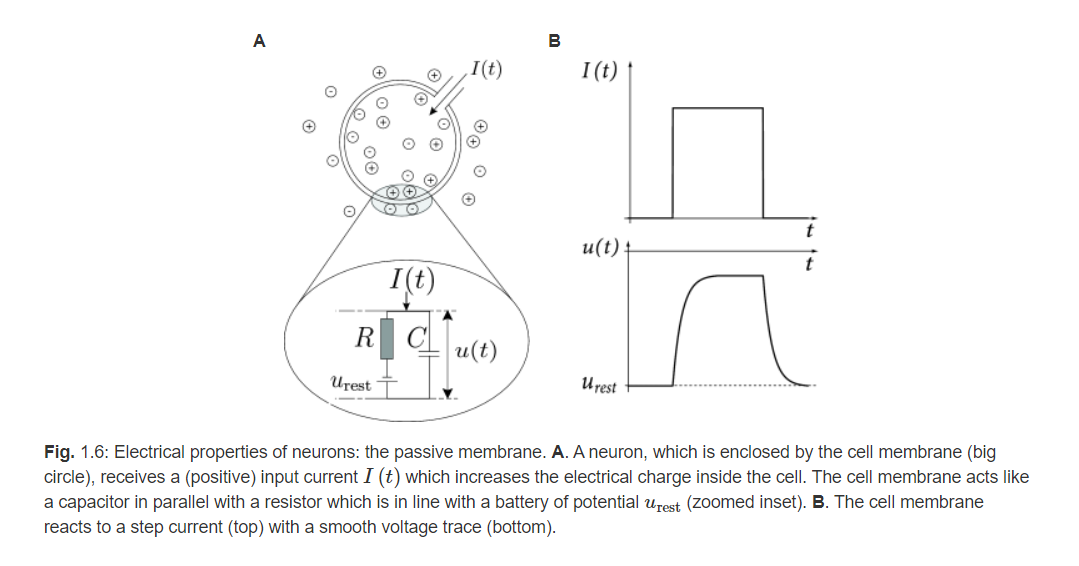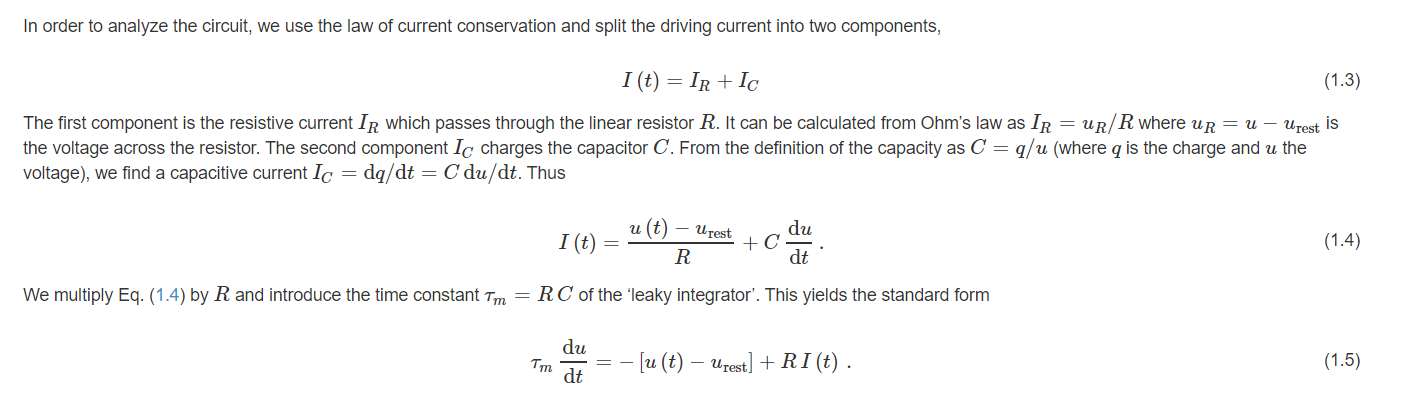二 代码
clear
close all

C = 0.2;          % capacitance in nF
R = 100;          % resitance in megaohm
dt = 0.01;        % integration time step in milliseconds
dur = 0.3;        % simulation duration in sec
Vthresh = -60;    % threshold in mV
EL = -70;         % leakage reversal potential in mV
Vreset = -70;     % reset voltage in mV
V0 = -70;         % initial condition in mV

tref = 3;         % refractory period in msec
Ij = 0.15;          % injected current in nA

dur = dur*1000;      % simulation duration in msec
niter = floor(dur/dt)+1;    % number of iterations
V = EL;                     % initial condition
spikes = zeros(1,niter);    % vector for storing binary spike train
Vm = zeros(1,niter);        % vector for storing Vm
Vm(1) = V0;          % assign initial condition to first element of Vm
t_vector = 0:dt:(length(Vm)-1)*dt;  % vector of time values
tcounter = tref;            % counter for how long we have been refracted,
% it is not refracted when we start
taum = R*C;                 % time constant in msec

for idx =  2 : niter    % loop for number of iterations
% check if we are refracted
if tcounter < tref
V = Vreset;
tcounter = tcounter + dt;   % update refractory counter
else     % we integrate as before
dVdt =(1/taum) .* ((EL - V) + R * Ij);
V = V + dt .* dVdt;
end
% check if spiking
if V > Vthresh
spikes(idx) = 1;
V = Vreset;
tcounter = 0;   % reset refractory counter
end
Vm(idx) = V;
end

plot(t_vector,Vm);
xlabel('time (ms)');
ylabel('V_m (mV)');

运行结果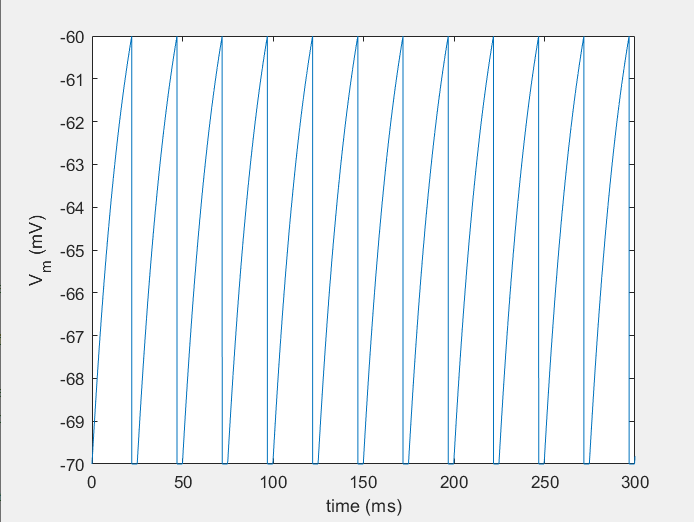三 参考文献
SNN系列｜神经元模型篇(4) LIF Neuronal Dynamics(book)
展开全文• 自己从官网和手册整理的例程文件合辑，也有自己添加的注释 example 里是自己整理的四个介绍文档对应的程序以及官网例程对应的.ipynb文件 examples 里是官网提供镜像关于NEST仿真的例程 ...
• 脉冲神经网络 神经元模型-HH模型Hodgkin Huxley 神经元模型及实现一 原理1. 电路图2. 常微分方程二 代码实现-python版本1. 模拟离子通道2. 模拟膜电位三 参考 Hodgkin Huxley 神经元模型及实现 最近在接触脉冲神经...


脉冲神经网络 神经元模型-HH模型
Hodgkin Huxley 神经元模型及实现一 原理1. 电路图2. 常微分方程
二 代码实现-python版本1. 模拟离子通道2. 模拟膜电位
三 代码实现-matlab版本四 参考

Hodgkin Huxley 神经元模型及实现
最近在接触脉冲神经网络相关知识，如有错误，请多多指教！
一 原理
1. 电路图Hodgkin-Huxley(HH)模型可以看作是rc电路模型的扩展，该模型除了泄漏通道外还包含钠(Na)和钾(K)通道。离子通道被建模为与电池串联的电阻器。电池表示特定离子的平衡电势，电阻器反映通道对特定离子的渗透性。与泄漏通道的固定电阻相比，Na和K通道的电阻有自己的动态特性，这取决于跨膜的电压。 更精确地说，对于给定的膜电位，每个通道都有相应的开启和关闭速率。这些通道的打开还是关闭是由霍奇金根据经验建立的函数和函数来描述的。这些和函数可以用在微分方程中来描述开放通道的比例如何随时间变化：

d

n

d

t

=

α

n

(

V

)

(

1

−

n

)

−

β

n

(

V

)

n

（

公

式

一

）

\frac{dn}{dt} = \alpha_n(V)(1-n) - \beta_n(V)n （公式一）

alpha_n和 beta_n描述打开通道的比例如何随时间变化

τ

n

=

1

α

n

+

β

n

(

公

式

二

)

\tau_n = \frac{1}{\alpha_n + \beta_n} (公式二)

表示多久通道才会打开

n

∞

=

α

n

α

n

+

β

n

(

公

式

三

)

n_{\infty} = \frac{\alpha_n}{\alpha_n + \beta_n}(公式三)

表示通道打开的概率
2. 常微分方程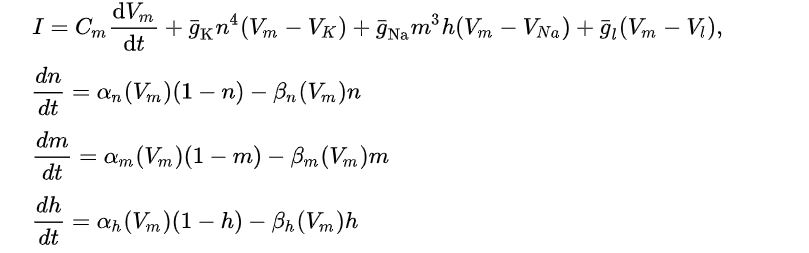由于HH模型需要使用4个常微分方程进行表示模型，故计算较为复杂，很难进行大规模仿真。但其精确地描绘出膜电压的生物特性，能够很好地与生物神经元的电生理实验结果相吻合
二 代码实现-python版本
import numpy as np
import matplotlib.pyplot as plt
%matplotlib inline

1. 模拟离子通道
# 离子通道被建模为与电池串联的电阻器。电池表示特定离子的平衡电势，电阻器反映通道对特定离子的渗透性
V = np.arange(-80.0,80,0.01) # [mV]

#n用来限制k离子的通透性，m和h用来限制na离子，因为na离子含有失活门，即使通道本身是打开的，如果失活门阻塞通道，离子电流也不能流动。
alpha_n = 0.01 *(10-V)/(np.exp((10-V)/10)-1)
alpha_m = 0.1*(25-V)/((np.exp((25-V)/10))-1)
alpha_h = 0.07 * np.exp(-(V/20))

beta_m = 4* (np.exp(-V/18))
beta_h = 1/(np.exp((30-V)/10)+1)
beta_n = 0.125 * np.exp(-(V/80))

#公式二、公式三实现
tau_n = 1/(alpha_n + beta_n)
inf_n = alpha_n*tau_n

tau_m = 1/(alpha_m + beta_m)
inf_m = alpha_m*tau_m

tau_h = 1/(alpha_h + beta_h)
inf_h = alpha_h*tau_h

#绘图
plt.clf()
plt.subplot(1,2,1)
plt.plot(V,inf_n)
plt.plot(V,inf_m)
plt.plot(V,inf_h)
plt.xlabel('Voltage (mV)')
plt.subplot(1,2,2)
plt.plot(V,tau_n)
plt.plot(V,tau_m)
plt.plot(V,tau_h)
plt.title('Time constants')
plt.xlabel('Voltage (mV)')
plt.legend(['n','m','h'])

运行结果如下图：由图可知，K离子选通变量

n

n

表明在平衡电位，大多数K趋于关闭。随着膜电位的增加，通道开始打开，但有一定的延迟。对于钠通道，当膜电位增加时，m值也增加，时间常数表明变化几乎是瞬时的（值很小）。然而，

h

h

趋于下降，这说明，在相对高的电压下，许多钠通道会打开，但大多数通道是失活的，因此几乎不会有离子电流。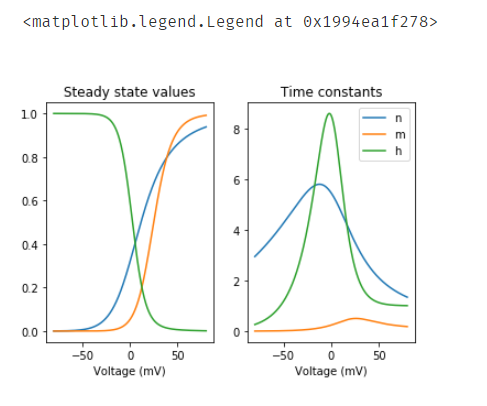2. 模拟膜电位

−

C

m

d

V

d

t

=

g

L

(

V

−

E

L

)

+

g

K

n

4

(

V

−

E

K

)

+

g

N

a

m

3

h

(

V

−

E

N

a

)

−

I

e

x

t

e

r

n

（

公

式

四

）

-C_m\frac{dV}{dt} = g_L(V-E_L) + g_Kn^4(V-E_K) + g_{Na}m^3h(V-E_Na) - I_{extern} （公式四）

# 参数定义 可参考
# 平衡电位
E_Na = 115.0   # [mV]
E_K  = -12.0   # [mV]
E_L  = 10.6    # [mV]

# 最大电导
g_Na = 120.0   # [mS]
g_K  = 36.0    # [mS]
g_L  = 0.3     # [mS]

dt = 0.01      # [ms]
T = 40         # [ms]
t = np.arange(0,T,dt)

V = np.zeros(len(t))
n = np.zeros(len(t))
m = np.zeros(len(t))
h = np.zeros(len(t))

I_E = 0.0
V = 0.0
h = 0.59
m = 0.05
n = 0.31

# 在10ms是注入10mA的电流，然后15ms再次置电流为0
for i in range(1,len(t)):
if i == 1000:
I_E = 10.0
if i == 1500:
I_E = 0.0

# Calculate the alpha and beta functions （代码同上）
alpha_n = (0.1 - 0.01*V[i-1]) / ( np.exp(1   - 0.1*V[i-1]) - 1)
alpha_m = (2.5 - 0.1 *V[i-1]) / ( np.exp(2.5 - 0.1*V[i-1]) - 1)
alpha_h = 0.07*np.exp(-V[i-1]/20.0)

beta_n = 0.125*np.exp(-V[i-1]/80.0)
beta_m = 4.0 * np.exp(-V[i-1]/18.0)
beta_h = 1 / ( np.exp(3 - 0.1*V[i-1]) + 1)

# Calculate the time constants and steady state values
tau_n = 1.0/(alpha_n + beta_n)
inf_n = alpha_n*tau_n

tau_m = 1.0/(alpha_m + beta_m)
inf_m = alpha_m*tau_m

tau_h = 1.0/(alpha_h + beta_h)
inf_h = alpha_h*tau_h

# 更新n,m,h
n[i]  = (1-dt/tau_n)*n[i-1] + (dt/tau_n)*inf_n
m[i]  = (1-dt/tau_m)*m[i-1] + (dt/tau_m)*inf_m
h[i]  = (1-dt/tau_h)*h[i-1] + (dt/tau_h)*inf_h

# 公式四
# 更新膜电位方程
I_Na = g_Na*(m[i]**3)*h[i]  * (V[i-1]-E_Na)
I_K  = g_K *(n[i]**4)       * (V[i-1]-E_K)
I_L  = g_L                  * (V[i-1]-E_L)

#dv = -(I_Na + I_K + I_L - I_E)
dv = I_E - (I_Na + I_K + I_L)
V[i]  = V[i-1] + dv*dt

plt.clf()
plt.subplot(1,3,1)
plt.plot(t,V)
# 膜电位变化
plt.title('Membrane potential')
plt.subplot(1,3,2)
plt.plot(t,n)
plt.plot(t,m)
plt.plot(t,h)
plt.title('Gating variables')
plt.legend(['n','m','h'])
plt.subplot(1,3,3)
plt.plot(t,g_Na*h * m**3)
plt.plot(t,g_K*n**4)
plt.title('Ionic currents')
plt.legend(['Na','K'])

运行结果如下图：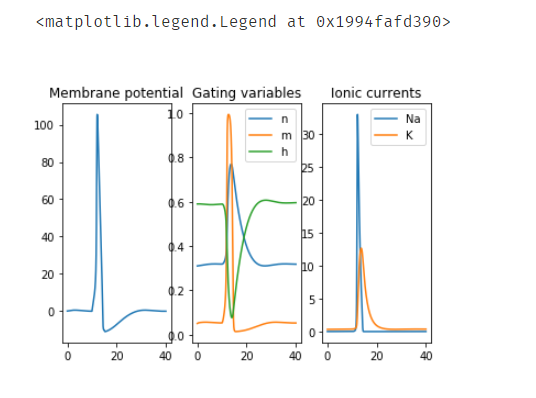三 代码实现-matlab版本
%% Basic Hodgkin-Huxley Implementation
% Equations from Hodgkin, Huxley, J. Physiol (1952) 117, 500-544

clear;
figure(1);clf;

dt = 0.001;
t = 0:dt:40;
% Nernst Potentials
Ena = 115; Ek = -12; El = 10.6;

% Maximum Conductances
gna = 120; gk = 36; gl = 0.3;

% Membrane Capacitance
C = 1;

% 离子通道打开
an = @(u) (0.1-0.01*u)/(exp(1-0.1*u)-1);
am = @(u) (2.5-0.1*u)/(exp(2.5-0.1*u)-1);
ah = @(u) 0.07*exp(-u/20);

% 离子通道关闭
bn = @(u) 0.125*exp(-u/80);
bm = @(u) 4*exp(-u/18);
bh = @(u) 1/(exp(3-0.1*u)+1);

% DEFINE FORMULAE FOR STEADY STATE GATE ACTIVATIONS

m_inf = @(u) am(u) / ( am(u) + bm(u) );
n_inf = @(u) an(u) / ( an(u) + bn(u) );
h_inf = @(u) ah(u) / ( ah(u) + bh(u) );

% INITIALIZE STATE VARIABLES

m = 0*t + m_inf(0);
n = 0*t + n_inf(0);
h = 0*t + h_inf(0);
v = 0*t;

% DEFINE STIMULUS STRENGTH, DURATION, & DELAY

tSTIM_START = 10;
tSTIM_DUR = 1;
STIM_STRENGTH = 10;

% DEFINE MISC

inRange = @(x,a,b) (x>=a) & (x<b);

% MAIN LOOP

for i = 1:length(t)-1

% extract membrane voltage
u = v(i);

% solve for membrane currents
Ik  = gk  * n(i)^4      * (u - Ek);
Ina = gna * m(i)^3*h(i) * (u - Ena);
Il  = gl  *               (u - El);
Imem = Ik + Ina + Il;

% determine stimulus current, if any
Istim = 0;
if inRange( t(i) , tSTIM_START , tSTIM_START+tSTIM_DUR)
Istim = STIM_STRENGTH;
end

% define the state variable derivatives
dv = (Istim - Imem)/C;
dm = am(u) * (1-m(i)) - bm(u) * m(i);
dh = ah(u) * (1-h(i)) - bh(u) * h(i);
dn = an(u) * (1-n(i)) - bn(u) * n(i);

% use forward euler to increment the state variables
v(i+1) = v(i) + dv*dt;
m(i+1) = m(i) + dm*dt;
h(i+1) = h(i) + dh*dt;
n(i+1) = n(i) + dn*dt;

end

% plot the results
plot(t,v);
axis([t(1) t(end) -20 120]);
xlabel('time (ms)');
ylabel('membrane voltage (mV)');

运行结果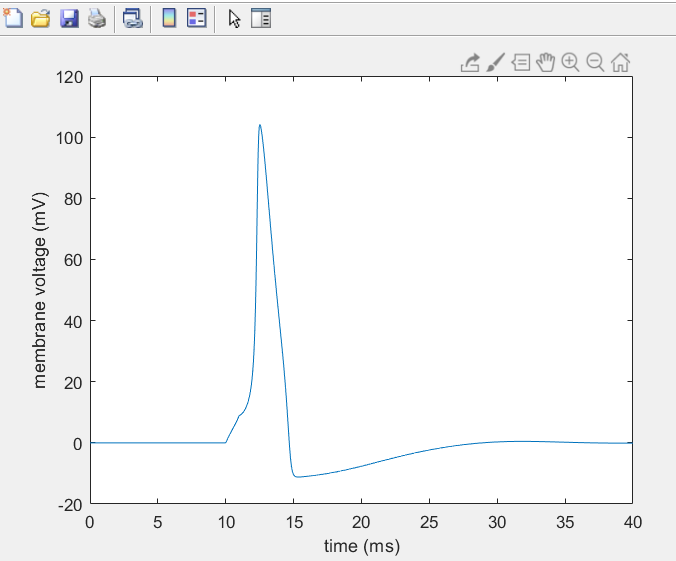四 参考
Hodgkin Huxleymatlab参考代码
展开全文• 1.1.1 脉冲神经网络神经元 为了去了解大脑是如何工作的，我们需要把结合实验学习动物和人类神经系统和大规模脑模型的数值研究结合起来。突触其实就是神经元信息传递的关键部分，是前神经元的轴突和后神经元的树突相...
前段时间研查阅文献时的matlab方面的探索，这一部分主要参考文献为
 朱娟. 基于尖峰神经元模型的机器人学习方法研究 [D]; 南京理工大学, 2014.
1.1 模型建立
1.1.1 脉冲神经网络神经元
为了去了解大脑是如何工作的，我们需要把结合实验学习动物和人类神经系统和大规模脑模型的数值研究结合起来。突触其实就是神经元信息传递的关键部分，是前神经元的轴突和后神经元的树突相连接的部分，突触可以分为化学突触和电突触，在研究中化学突触要比电突触常见。当神经冲动到达突触前膜时，这时突触前膜上的Ca2+通道就会被其触发并且被打开，这时细胞外的Ca2+就会进入到突触前部，接着突触小泡向突触前膜移动并将内部的神经递质释放到突触间隙。有一部分的神经递质就会和突触后膜上相应的受体相结合，从而使受体相关的门控通道打开，相应的离子由通道进入突触后部，从而使突触后神经元的活动受到影响。突触信息的传递过程可归纳为如图：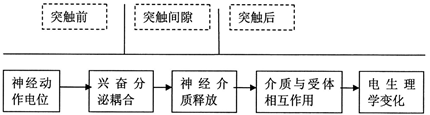突触信息传递的特点可归纳为：
(1)信息传递一般都有延时
(2)兴奋性、抑制性
(3)存在不应期
(4)可塑性：突触传递信息的强度是可以改变的，具有学习能力。可塑性是学习和记忆的基础。
(5)对信息的整合有两种方式，时间累加和空间累加。
(6)具有遗忘和疲劳
(7)信息传递是不可逆的：脉冲只能从突触前神经元传到突触后神经元。
朱娟等人[最前面提到的那篇]分析比较了第三代与之前的神经元模型，深入的对尖峰神经元的三种代表性模型进行仿真并比较各自的优缺点，在以较小的复杂度达到较高的生物学的真实性基础上选择了适合本文的Izhikevich模型，重点讨论了Izhikevich模型，对常见的6种尖峰进行仿真研究，深入了解了模型中各个参数的作用。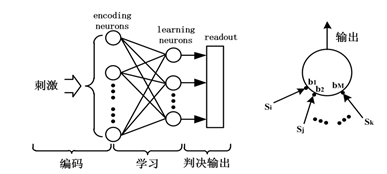门电路可以看成是计算机最基本的一个单元，故而神经元也就可以当作是神经系统的一个基本单元。但是神经元有的功能却远远比门电路来的复杂。目前神经元模型有一百多种，表现出了神经元的各种特性(时空总和、阀值、不应期、适应性以及可塑性)。
由于研究的最终目的有所不同，所以对于神经元模型的研究方向也就不同主要有两点。一是，单神经元的研究，这时就希望尽最大努力去复现神经元本身的属性。二是，由神经元组成功能复杂的神经网络，所以用到的神经元数目比较多，为了研究的方便，我们要求神经元保留信号处理能力的前提下能够简化神经元模型。神经元模型的一些特点如下：
(1)神经元的输入有很多但是输出端口只有一个。
(2)神经元的阀值是一个定值。
(3)神经元是非时变的。
(4)神经元产生脉冲的条件：
1)每个兴奋型输入为+1表示，抑制性输入用-1表示，当输入的总和大于固定的阀值时神经元这时才会产生脉冲信号。
2)没有抑制性输入，只有兴奋型输入时，当输入总和超过阀值时，神经元才会产生脉冲。
下面着重介绍两种模型，漏积分模型和尖峰神经元模型仿真来研究为什么本文要采用尖峰神经元模型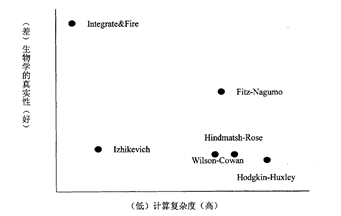(1)漏积分神经元模型
现在大部分神经网络中的神经元都采用漏积分模型来研究如式
​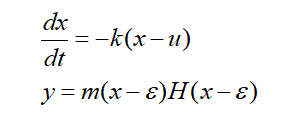其中x表示神经元的状态，u用来表示神经元的输入，y代表神经元的输出，k,m,e三者是模型的参数，H为阶跃函数。仿真设置：输入u为阶跃输入， k=1,m=1,e=0.2 得到输出曲线如所示：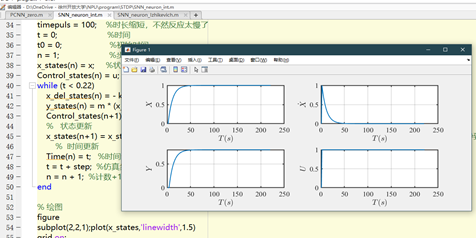(2)尖峰神经元模型
尖峰神经元模型种类很多这里选择Izhikevich模型如式来研究
​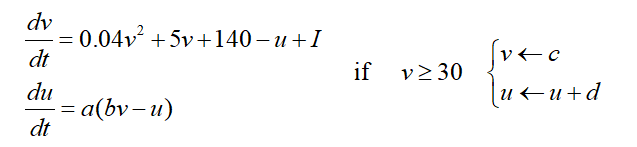v为膜电位，u表示恢复变量，膜电流则用I代表 a,b,c,d为参数。仿真设置：a=0.02,b=0.25,c=-65,I=10得到的响应如图所示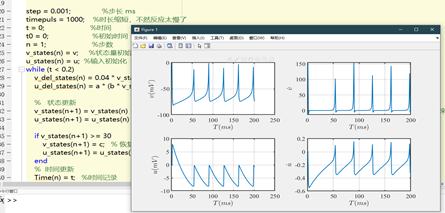可以看出漏积分神经元模型主要是将信息编码放在输出信号的幅值中。而尖峰神经元则不是，它是将信息编码在神经元的动作电位的频率中，因此这种模型对于模拟真实神经元的放电特性更加逼真
1.1.2 几种代表性的尖峰神经元模型的比较
尖峰神经元模型最早的提出者是Hodgkin和Huxley。根据脉冲神经元的响应模式和生化模型，学者们提出了一些用于模拟脉冲神经元行为的数学模型，一般将提出三种具体的脉冲神经元模型——Itegrate&Fire、Izhikevich以及Hodgkin-huxley三种模型。并对这三种模型的表达式进行对比和仿真研究：
(1) Integrate&Fire模型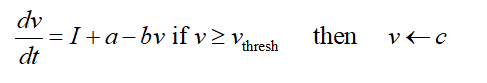式中 v为膜电位， I为膜电流， a,b,c,v_为参数。IF模型通过式可以看出是一维方程而且只有一个v变量，它主要通过线性微分方程来描述神经元，它的阀值以及复位机制均是固定的。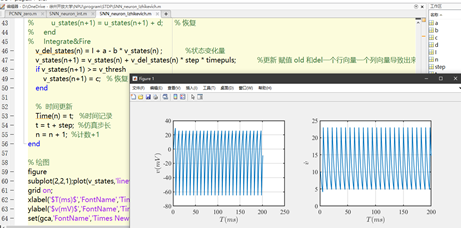结论：通过仿真可以发现IF模型的神经元没有不应期(所谓的不应期是指在神经元恢复到静息状态前，它不能通过发放动作电位来对刺激做出一定的响应，这段恢复时间被称为神经元的不应期，一般为 )，因而不够精确不能展现神经元最基本的特性。而且通过改变参数不会出现神经元的峰放电以及簇放电等特性。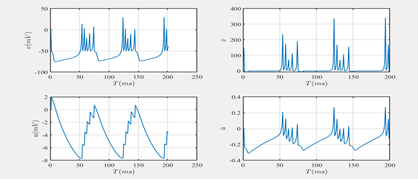(2)Izhikevich模型
模型如式(2．2)所示，仿真参数设置：a=0.02,b=0.25,c=-65,I=10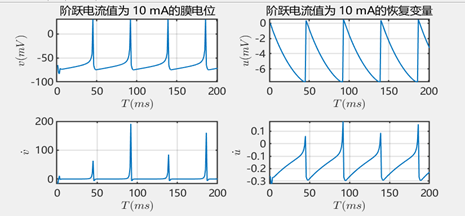结论：通过图可以看出神经元模型存在不应期，而且随着电流强度的变大尖峰频率也在增加。当刺激超过阀值时神经元会产生始终响应，使兴奋与静息交替产生，而且兴奋的周期远远小于静息的周期，这一点与真实神经系统受到外界刺激时作出的响应相符合。
(3)Hodgkin-Huxley模型其中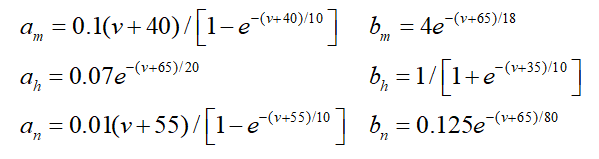理解就好，剩下的可以看文献
最终结论：IF只有1个变量，数值模拟比较简单，所以它适合于数学分析，但是却无法反应相位刺激、爆发式点火和回荡反应等行为。实质上这种模型不能算作真正的脉冲神经元模型，当膜电位到达阀值时，神经元产生的脉冲都是假想的。H-H模型含有4个变量，参数最为丰富，所以它对于模型真实神经元的某些特性(例如，对于电流脉冲的响应以及阀值效应的产生)是很精确的。所以我们一般用其研究单个的神经细胞的行为与特性，对于很多的神经元构成的神经系统却很少使用这种模型。Izhikevich模型含有2个变量，它在计算复杂度和生物学上的真实性两者间进行了折中。
因为我们要发展由尖峰神经元组成的这种大规模的脑模型，我们就必须要做到两个看似相互排斥的要求间的折中：单一神经元模型必须是简单的计算，但真正的生物神经元有产生丰富的放电模式能力的表现。使用H-H模型是最精确的，但是计算比较困难，因为我们仅仅可以实时的模拟极少数的神经元。相比之下，Integrate&Fire模型计算速度最快，而Izhikevich模型结合了H-H模型生理学特性和Integrate&Fire模型计算简单特性。取决于四个参数，该模型再现了神经元已知类型的尖峰。Izhikevich对神经元不同输入发生的分叉分类，并揭示了神经系统复杂行为的非线性动力学机制。
6种神经元比较：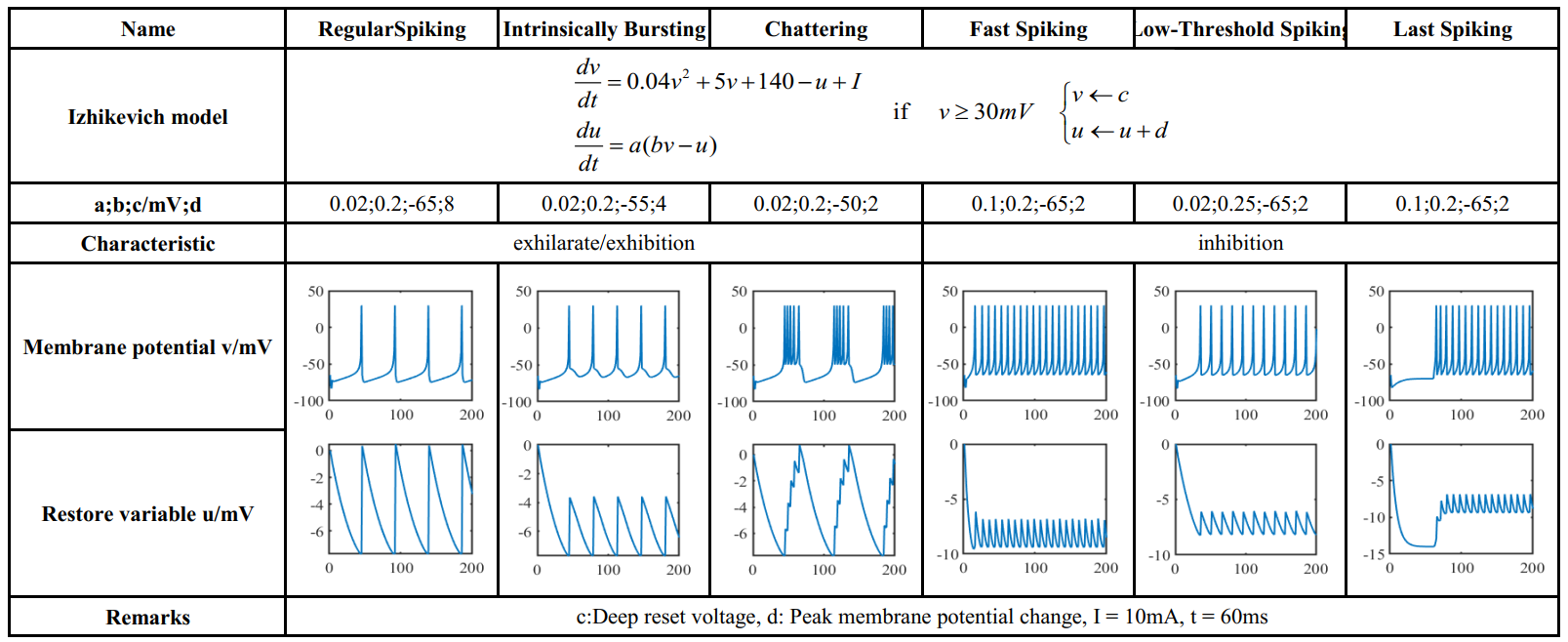通过图可以看出通过改变c,d参数可以使神经元实现簇放电。其中，u 和 v是无量纲变量， a,b,c,d是四个无量纲参数， v作为神经元的膜电位， u为神经元恢复变量，表示神经元膜上Na+通道关闭而K+通道打开，这时Na+的膜透性迅速下降而K+的膜透性却迅速增强，膜电压恢复到静息电位的过程。当神经元产生动作电位，此时膜电位v达到峰值30mV时，膜电位和恢复变量将按照式2变化。 I是突触电流，即注入电流。模型各个参数的作用：
a：其实是一个时间因子主要用来反映恢复变量u，而u的恢复速度就是由a决定的，a越小表示u恢复的越快，尖峰脉冲的频率也就越高，a的一个典型值为0.02。
b：恢复变量对于v波动时的敏感度就是由b反映的，但是有一个条件就是v必须在阀值下。b 越大，u和v的联系就越强，阀值下的震荡就越强烈并且产生尖峰序列的阀值就越小，典型值为0.2。
c：在动作电位产生后，膜电位恢复值v的大小条件是由快速高阀值K+电导引起，典型值为-65mV 。
d：动作电位产生后由K+ 电导和Na+电导引起的恢复变量u的值。
0.04v*v+5v=140这一部分是通过对神经元的尖峰过程动态拟合而来的，膜电位的单位为 ，时间的单位为mV 。细胞膜的静息电位一般为-70~-60mV ，具体情况则要由参数b决定。在大多数的神经细胞中，一般阀值是不固定的，它受到前面神经细胞状态的影响。阈值电位的变化有个范围一般是-55~-40mV。
神经元模型介绍了动态突触和主动树突结合功能的优点。神经元能够通过隐式“延迟”机制系统最大化其响应并检测特定的时间序列。这些机制基于对突触动力学和神经活动特性产生的突触后电位的调制。学习算法会调整“延迟”机制，以使它们针对输入尖峰序列的特定时间序列产生最大的响应（就躯体处的膜电位而言）。
1.2 SNN仿真
这一部分的结果当时用了几天的时间调出来，但是最后也没有用到（需要的计算量其实并不小），仅仅提供仿真思路参考，也没法用代码解释，放几张图之后随缘上传源文件吧。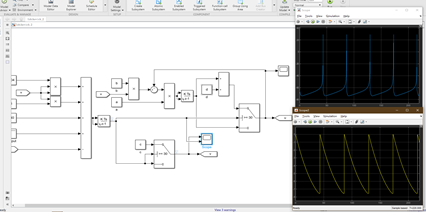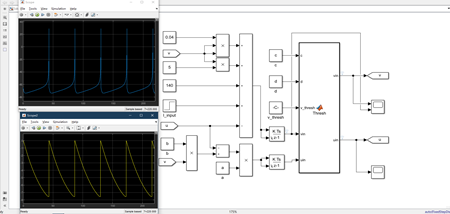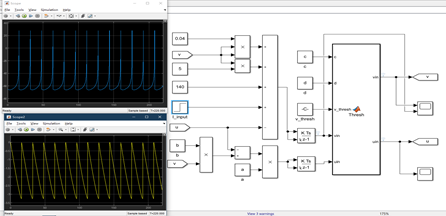六个模块对应六种模型：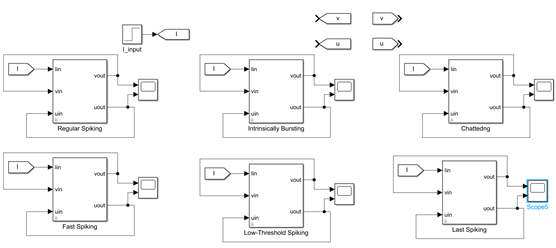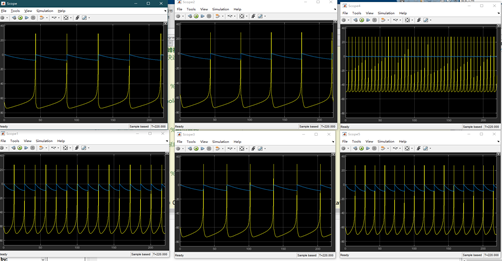源代码：绘图部分因为其他需要注释掉了微分量，如果需要自行修改即可。
% 脉冲神经网络matlab实现
% 朱娟. 基于尖峰神经元模型的机器人学习方法研究 [D]; 南京理工大学, 2014.
% 神经元及突触模型仿真
% 2020年10月10日 Jonny Su
clc;
% close all;
clear all;
cd ('D:\OneDrive - 徐州开放大学\NPU\program\STDP')

%%   Simulation parameters
v = -65;                  %初始膜电位
u = 0;                      %初始恢复变量
I = 10;                  %膜电流 影响放电频率
v_thresh = 30;               %电位阈值

step = 0.001;               %步长 ms
timepuls = 10000;    %时长缩短，不然反应太慢了
t = 0;                      %计时器
t0 = 0.002;                     %初始输入电流阶跃时间
n = 1;                      %步数

v_states(n) = v;     %状态量初始化
u_states(n) = u;  %输入初始化
%%  Izhikevich尖峰神经元模型中，兴奋型皮层细胞可以分为以下三类：

% RS(Regular spiking)常规性尖峰
% a = 0.02;                  %模型参数
% b = 0.2;                  %模型参数
% c = -65;                  %模型参数
% d = 8;                  %模型参数

% IB(intrinsically bursting)本征性爆发尖峰神经元
% a = 0.02;                  %模型参数
% b = 0.2;                  %模型参数
% c = -55;                  %模型参数
% d = 4;                  %模型参数

% CH(chattedng)震颤式尖峰神经元
% a = 0.02;                  %模型参数
% b = 0.2;                  %模型参数
% c = -50;                  %模型参数
% d = 2;                  %模型参数

%%  Izhikevich尖峰神经元模型中，抑制性脑皮层细胞也可以分为以下三类：
% FS(fast spiking)快速式尖峰神经元
% a = 0.1;                  %模型参数
% b = 0.2;                  %模型参数
% c = -65;                  %模型参数
% d = 2;                  %模型参数

% LTS(Low-Threshold spiking)低阀值尖峰神经元
% a = 0.02;                  %模型参数
% b = 0.25;                  %模型参数
% c = -65;                  %模型参数
% d = 2;                  %模型参数

% LS(Last spiking)延时尖峰神经元  60ms
a = 0.1;                  %模型参数
b = 0.2;                  %模型参数
c = -65;                  %模型参数
d = 2;                  %模型参数
t0 = 0.06;                     %延时阶跃时间
while (t < 0.2)
%     Izhikevich
v_del_states(n) = 0.04 * v_states(n) * v_states(n) + 5 * v_states(n) +140 - u_states(n) + I * stepfun(t,t0);            %状态变化量
u_del_states(n) = a * (b * v_states(n) - u_states(n));     %状态量初始化

%   状态更新
%     v_states(n+1) = v_states(n) + v_del_states(n) * step * timepuls;           %更新 赋值 old 和del一个行向量一个列向量导致出来是矩阵
%     u_states(n+1) = u_states(n) + u_del_states(n) * step * timepuls;           %控制量
%     直接什么都不乘完全是合理的，步长少了增量也少了，自然没有必要再缩减几个量级了。
v_states(n+1) = v_states(n) + v_del_states(n);           %更新 赋值 old 和del一个行向量一个列向量导致出来是矩阵
u_states(n+1) = u_states(n) + u_del_states(n);           %控制量
if v_states(n+1) >= v_thresh        %没有起到作用感觉
v_states(n) = v_thresh;   % 弥补步长导致没法还原步长的错误，不太合理
v_states(n+1) = c;   % 恢复
u_states(n+1) = u_states(n+1) + d;      % 恢复
end
%     Integrate&Fire
%     v_del_states(n) = I + a - b * v_states(n) ;            %状态变化量
%     v_states(n+1) = v_states(n) + v_del_states(n) * step * timepuls;           %更新 赋值 old 和del一个行向量一个列向量导致出来是矩阵
%     if v_states(n+1) >= v_thresh
%         v_states(n+1) = c;   % 恢复
%     end

%  时间更新
Time(n) = t;   %时间记录
t = t + step;  %仿真步长
n = n + 1;  %计数+1
end

% 绘图
figure
subplot(2,1,1);plot(v_states,'linewidth',1.5)
% title(['阶跃电流值为 ',num2str(I),' mA的膜电位'],'FontSize',14);
% grid on;
% xlabel('$T(ms)$','FontName','Times New Roman','FontSize',14,'Interpreter','latex');
% ylabel('$v(mV)$','FontName','Times New Roman','FontSize',14,'Interpreter','latex');
% set(gca,'FontName','Microsoft YaHei UI','FontSize',14,'linewidth',1.5);
set(gca,'FontName','Times New Roman','FontSize',14,'linewidth',1.5);
% subplot(2,2,3);plot(v_del_states,'linewidth',1.5)
% grid on;
% xlabel('$T(ms)$','FontName','Times New Roman','FontSize',14,'Interpreter','latex');
% ylabel('$\dot{v}$','FontName','Times New Roman','FontSize',14,'Interpreter','latex');
% set(gca,'FontName','Microsoft YaHei UI','FontSize',14,'linewidth',1.5);

subplot(2,1,2);plot(u_states,'linewidth',1.5)
% title(['阶跃电流值为 ',num2str(I),' mA的恢复变量'],'FontSize',14);
% grid on;
% xlabel('$T(ms)$','FontName','Times New Roman','FontSize',14,'Interpreter','latex');
% ylabel('$u(mV)$','FontName','Times New Roman','FontSize',14,'Interpreter','latex');
% set(gca,'FontName','Microsoft YaHei UI','FontSize',14,'linewidth',1.5);
set(gca,'FontName','Times New Roman','FontSize',14,'linewidth',1.5);
% subplot(2,2,4);plot(u_del_states,'linewidth',1.5)
% grid on;
% xlabel('$T(ms)$','FontName','Times New Roman','FontSize',14,'Interpreter','latex');
% ylabel('$\dot{u}$','FontName','Times New Roman','FontSize',14,'Interpreter','latex');
% set(gca,'FontName','Microsoft YaHei UI','FontSize',14,'linewidth',1.5);

结尾
其实这几个月还是没闲着一直在做工作，平时不咋记得码博客了，最近网站也出了问题，莫名会跳转到其他网站去，也没有时间收拾了。年初的论文不知道是第几次被拒了，现在又在准备另一篇，这部分内容算是里面的一小部分，希望能共同学习，一切都会好起来的。 今年可真快，加油。
欢迎访问我的个人网站 （目前死机，明年也不一定续）https://www.josu.top/
展开全文matlab
• ## 脉冲神经网络（SNN）概述

万次阅读 多人点赞 2019-06-18 08:37:15
主要讨论脉冲神经网络的拓扑结构、信息的脉冲序列编码方法、脉冲神经网络的学习算法和进化方法等。 一、脉冲神经网络的拓扑结构 同传统的人工神经网络一样，脉冲神经网络同样分为三种拓扑结构。它们分别是前馈型...
• ## 脉冲神经元模型

万次阅读 2018-05-28 20:45:18
脉冲神经元模型传统的人工神经元模型主要包含两个功能，一是对前一层神经元传递的信号计算加权和，二是采用一个非线性激活函数输出信号。 前者用于模仿生物神经元之间传递信息的方式，后者用来提高神经网络的非线性...
• 最近了解了一下脉冲神经网络(Spiking Netrual Network, SNN)在Event Camera的一些应用。在此稍作整理。由于本人对神经网络了解不多，难免存在理解错误，欢迎大家积极指正。转载请注明出处 1. 脉冲神经网络 ...
• 一. 时钟驱动模拟策略 1. 神经元模型的数值计算方法 一般来说，大多数生物系统建立的模型是微分方程形式，并且这些方程不能简单地通过...对于脉冲神经元模型的数值计算，一般采用欧拉或龙格-库塔等数值方法进行近...
• ## 脉冲神经网络

千次阅读 2018-05-07 09:28:00
脉冲神经网络 （SNN-Spiking Neuron Networks) 经常被誉为第三代人工神经网络。旨在弥合神经科学和机器学习之间的差距，使用最拟合生物神经元机制的模型来进行计算。第一代神经网络是感知器，它是一个简单的神经元...
• 脉冲神经网络被称作是第三代神经网络，生物可信度更高，在近年来兴起的类脑科学研究，SNN也一直占据着核心地位。并且在性能相近的情况下，基于脉冲神经网络制成的芯片相较于人工神经网络功耗更低，稳定性、鲁棒性...python 机器学习 人工智能
• 主要讨论脉冲神经网络的拓扑结构、信息的脉冲序列编码方法、脉冲神经网络的学习算法和进化方法等。 一. 脉冲神经网络的拓扑结构 同传统的人工神经网络一样，脉冲神经网络同样分为三种拓扑结构。它们分别是前馈型脉冲...
• 这些脚本实现了 Bourdoukan R、Barrett DGT、Machens CK、Deneve S (2012) 提出的尖峰神经网络模型。 学习基于最佳尖峰的表示，神经信息处理系统 (NIPS) 的进展 25。这是论文的摘要 神经网络如何学习表示信息？ 在...MATLAB
• ## 脉冲神经网络学习笔记（综述）

万次阅读 多人点赞 2017-11-26 16:03:05神经网络 算法
• 参考资料： 类脑运算--脉冲神经网络（Spiking Neural Network）叙述：https://blog.csdn.net/Yannan_Strath/article/details/105761023 脉冲神经网络的五脏六腑：...脉冲神经元模型 1.IF neuron(int
• 生物神经元结构及脉冲发放 生物神经系统的信号是以细胞膜的电位变化来传导的。神经元细胞膜内外的电位有差别，外正内负，70-80mv。周围环境和内部变化可以引起膜电位的高低变化。但是这类变化当幅度不大时只是...
• ## SNN综述(1)：深度脉冲神经网络

千次阅读 多人点赞 2020-07-02 09:08:11
生物可解释的脉冲神经网络综述 作者：Aboozar Taherkhani, Ammar Belatreche, Yuhua Li, Georgina Cosma, Liam P. Maguire, T.M. McGinnity 译者：TianlongLee 时间：2020 原文链接：A review of learning in ...神经网络 深度学习
• 它们分别是前馈型脉冲神经网络（feed-forward spiking neural network）、递归型脉冲神经网络（recurrent spiking neural network）和混合型脉冲神经网络（hybird spiking neural network）。 学习是人工智能领域的...SNN 深度学习
• 为求解电晕电流的通用数学模型,利用人工神经网络能以任意精度逼近任意函数的能力,设计了2层BP神经网络,分别对实测的具有双指数函数、Gaussian函数及不规则脉冲形式的电晕电流进行拟合。结果表明,当神经元数目取5~10...
• Biologically Plausible Spiking Neural Networks 作者：Aboozar Taherkhani, Ammar Belatreche, Yuhua Li, Georgina Cosma, Liam P. Maguire, T.M. McGinnity 译者：TianlongLee ...脉冲神经网络根据类脑智能 SNN 模式识别
• 现代人工智能还难以在边缘设备广泛应用，而脉冲神经网络（Spiking neural network, SNN）有望突破造成这一困境的主要难题：基于深度学习的当前 SOTA 大型人工神经网络（ANN）的能量消耗。 尤其是卷积神经网络...人工智能 计算机视觉 机器学习 深度学习
• CoDi 是一种用于尖峰神经网络 (SNN) 的元胞自动机 (CA) 模型。 CoDi 是 Collect and Distribute 的首字母缩写，指的是神经网络中的信号和尖峰。 CoDi 使用为三维空间修改的冯诺依曼邻域； 每个单元查看它的六个正交...C++...